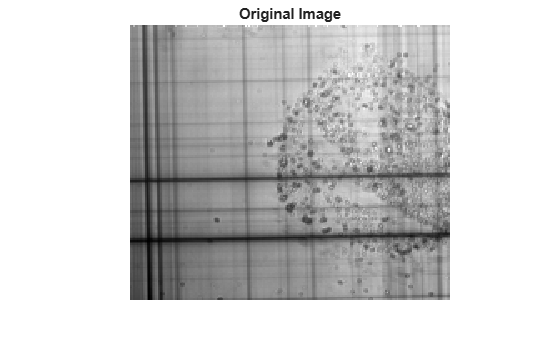Documentation

## Detect Lines Using the Radon Transform

This example shows how to use the Radon transform to detect lines in an image. The Radon transform is closely related to a common computer vision operation known as the Hough transform. You can use the radon function to implement a form of the Hough transform used to detect straight lines.

### Compute the Radon Transform of an Image

Read an image into the workspace. Convert it into a grayscale image.

I = fitsread('solarspectra.fts'); I = rescale(I);

Display the original image.

figure imshow(I) title('Original Image')Compute a binary edge image using the edge function. Display the binary image returned by the edge function.

BW = edge(I); figure imshow(BW) title('Edges of Original Image')Calculate the radon transform of the image, using the radon function, and display the transform. The locations of peaks in the transform correspond to the locations of straight lines in the original image.

theta = 0:179; [R,xp] = radon(BW,theta);

Display the result of the radon transform.

figure imagesc(theta, xp, R); colormap(hot); xlabel('\theta (degrees)'); ylabel('x^{\prime} (pixels from center)'); title('R_{\theta} (x^{\prime})'); colorbarThe strongest peak in R corresponds to $\theta =1$ degree and x' = -80 pixels from center.

### Interpreting the Peaks of the Radon Transform

To visualize this peak in the original figure, find the center of the image, indicated by the blue cross overlaid on the image below. The red dashed line is the radial line that passes through the center at an angle $\theta =1$ degree. If you travel along this line -80 pixels from center (towards the left), the radial line perpendicularly intersects the solid red line. This solid red line is the straight line with the strongest signal in the Radon transform.To interpret the Radon transform further, examine the next four strongest peaks in R.

Two strong peaks in R are found at $\theta =1$ degree, at offsets of -84 and -87 pixels from center. These peaks correspond to the two red lines to the left of the strongest line, overlaid on the image below.

Two other strong peaks are found near the center of R. These peaks are located at $\theta =91$ degrees, with offsets of -8 and -44 pixels from center. The green dashed line in the image below is the radial line passing through the center at an angle of 91 degrees. If you travel along the radial line a distance of -8 and -44 pixels from center, then the radial line perpendicularly intersects the solid green lines. These solid green lines correspond to the strong peaks in R.The fainter lines in the image relate to the weaker peaks in R.